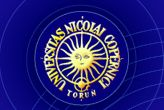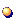HOME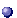DescriptionPrerequisitesIntroductionMereologyBasicsGrzegorczyk's systemGeometry of solidsPoint-free metric spacesBibliography24th European Summer School in Logic, Language and Information Point-free geometry and topology An introductory course Lecturer: dr Rafał Gruszczyński (Department of Logic, NCU in Toruń)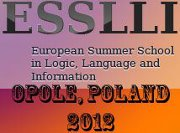A brief description of the course

The main purpose of the course is to familiarize an audience with various point-free approaches to geometry, topology and reasoning about space. By a point-free approach I mean such in which points are not assumed to be primitive objects of a theory, but are constructed from other entities (for example such that are more easily interpreted in the surrounding world).

Prerequisites

• lattices and Boolean algebras
• basic geometry and topology
• metric spaces

Part I: Introduction

The main purpose of the introductory part of the course is to set the correct perspective on the problem. First, it contains a refresher on fundamental notions from ordered sets theory and topology. Second, it explains the meaning of the notion point-free topology (geometry) we use in the whole course. Third, we remind what an ordinary point-based geometry is and how it can be done. Fourth, the origins of point-free approach to geometry are presented.

• slides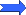• Part II: Mereology

The third part is fully devoted to mereology, a mathematical theory of part of relation, which will be further used as the main tool for development of various point-free theories of space. The most important notion of this part is that of a mereological sum (mereological set, collective set, fusion) of a group of objects. An axiomatization of mereology is given and the connection with the theory of Boolean algebras is explained. All the necessary material to grasp the ideas from this part of lectures can be found in (Pietruszczak, 2005).

• slides• Part III: Basic assumptions of point-free geometry

This part begins with an analysis of the Russellian perspective space from (Russell, 1914) We present Russell's arguments for point-free theory space which is motivated by properties of the perspective space. Afterward we move on to presentation of Stanisław Leśniewski view's on geometry. In the final stage we present the set of postulate and properties which may be called basic methodological and ontological assumptions of point-free theories of space.

• slides• Part IV: Grzegorczyk's system of topology

Part IV treats the system of point-free system of topology as constructed by Andrzej Grzegorczyk in (Grzegorczyk, 1960) with further analysis and emendations from (Biacino et al., 1996) and (Gruszczyński et al., 2011). In this part the following important notions are introduced and examined: of separation of regions, connection of regions, non-tangential inclusion of regions, quasi-separation structure and separation structure. By means of these we introduced some special sets of regions which are called pre-points. From these we construct points as filters generated by the former in separation structures. Further it is demonstrated that the notion of the open set is captured by the Grzegorczyk's theory and resulting topological space must always be Hausdorff.

• slides• Part V: Tarski's geometry of solids

Tarski's system of point-free geometry based on mereology was first presented in (Tarski, 1929) and further slightly modified and fully developed in (Gruszczyński et al., 2008). Tarski's idea was to enrich the axioms of mereology with one primitive notion of ball and series of definitions and axioms that let him introduce him the notion of point. Thanks to his simple but ingenious idea Tarski was also able to capture in his system the ternary notion of equidistance of points, thanks to which (and due to Mario Pieri's discovery from) he could talk about Euclidean geometry. This part of the lectures is devoted to presentation of aforementioned Tarski's ideas.

• slides• Part VI: Point-free metric spaces and verisimilitude theories

Point-free metric spaces as presented in (Gerla, 1990) are structures that are similar to metric spaces but such in which the notion of point is replaced with that of a region and a distance function on points is replaced with a distance between regions. An additional notion of the diameter of a region is also introduced. After the presentation the basics of the theory of point-free metric spaces we show an application of the theory o the solution of the problem from the philosophy of science — the Popperian idea of verisimilitude of scientific theories (Gerla, 2007).

• slides• Bibliography

• L. Biacino, G. Gerla “Connection structures: Grzegorczyk's and Whitehead's definitions of point”, Notre Dame Journal of Formal Logic, vol. 37, no. 3 (1996), pp. 431–439 (full text PDF)
• T. de Laguna “Point, line and surface as set of solids”, Journal of Philosophy, vol. 19 (1922), pp. 449–461
• G. Gerla “Pointless metric spaces”, Journal of Symbolic Logic, vol. 55, no. 1 (1990), pp. 207–219 (full text PDF)
• G. Gerla “Pointless geometries”, Handbook of Incidence Geometry, edited by F. Buekenhout and W. Kantor, North-Holland, Amsterdam (1995), pp. 1015–1031 (full text PDF)
• G. Gerla “Point-free geometry and verisimilitude of theories”, Journal of Philosophical Logic, vol. 36, no. 6 (2007), pp. 707–733 (full text PDF)
• R. Gruszczyński, A. Pietruszczak “Pieri's structures”, Fundamenta Informaticae, vol. 81, no. 1–3 (2007), pp. 139–154
• R. Gruszczyński, A. Pietruszczak “Full development of Tarski's geometry of solids”, The Bulletin of Symbolic Logic, vol. 14, no. 4 (2008), pp. 481–540 (full text PS)
• R. Gruszczyński, A. Pietruszczak “Space, points and mereology. On foundations of point-free Euclidean geometry”, Logic and Logical Philosophy, vol. 18, no. 2 (2009), pp. 145–188 (full text PDF)
• R. Gruszczyński, A. Pietruszczak “How to define mereological (collective) set”, Logic and Logical Philosophy, vol. 19, no. 4 (2010), pp. 309–328 (full text PDF)
• R. Gruszczyński, A. Pietruszczak “On Grzegorczyk's system of point-free topology”, unpublished paper (2011)
• Handbook of spatial logics, edited by M. Aiello, I. Pratt-Hartmann, J. van Benthem, Springer (2007)
• A. Pietruszczak Metamereology (in Polish), Nicolaus Copernicus University Press, Toruń (2000)
• A. Pietruszczak “Pieces of mereology”, Logic and Logic Philosophy , vol. 14, no. 2 (2005), pp. 211–234 (full text PDF)
• B. Russell Our knowledge of external world, The Open Court Publishing Company, 1914.
• A. Tarski “Les fondements de la gèometriè de corps”, pages 29–33 [in:] Księga pamiątkowa Pierwszego Polskiego Zjazdu Matematycznego, supplement to Annales de la Societè Polonaise de Mathèmatique}, Kraków 1929. English translation: “Fundations of the geometry of solid”, pages 24–29 [in:] Logic, Semantics, Metamathematics. Papers from 1923 to 1938, Oxford 1956.Beginning of Page